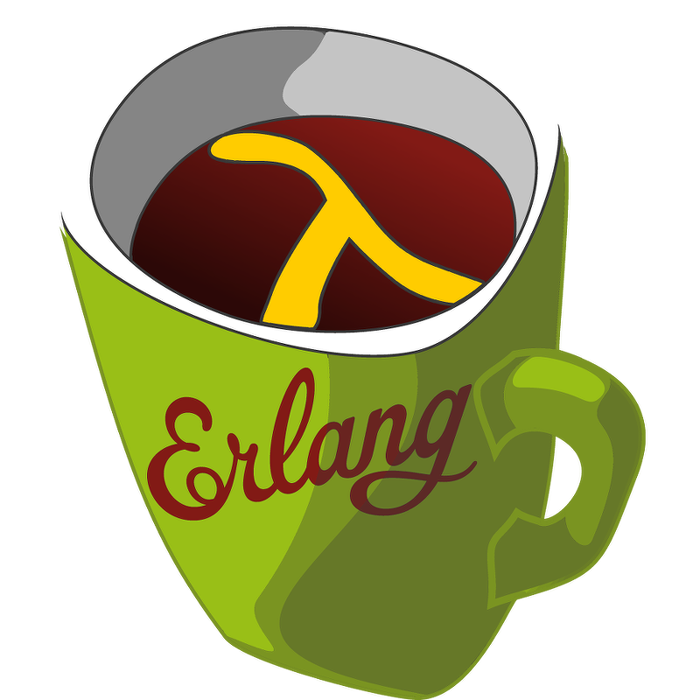Sometimes you need to evaluate a dynamic expression in LFE, one that has been created during the execution of the program. For example, imagine some other process has sent us an LFE expression which we need to evaluate.

This can be done with the function `eval/1`. As one might guess, `eval/1` evaluates its argument as an expression.

Here is an example:

``````> (eval '(+ 1 2 3))
6
> (eval '(calendar:is_leap_year 1200))
true``````

This works well if the expression is a literal value, but what if the expression has free variables for which we want to import values. For example we want get the value of `x` into `(+ 1 2 3 x)`:

``````> (set x 42)
42
> (eval '(+ 1 2 3 x))
exception error: #(unbound_symb x)``````

which is not quite what we had hoped. The solution is to build a `let` form where we can import values for the variables which need binding:

``````> (eval '(let ((x 42)) (+ 1 2 3 x)))
48``````

However, if the expression and values are not static then we must build the whole `let` expression to evaluate:

``````> (set x 42)
42
> (set expr '(+ 1 2 3 x))
(+ 1 2 3 x)
> (list 'let (list (list 'x x)) expr)
(let ((x 42)) (+ 1 2 3 x))
> (eval (list 'let (list (list 'x x)) expr))
48``````

Problem solved, now we can build expressions and import values into them. However, even with an expression as simple as this one building the structure can be quite complex. Fortunately, to the rescue comes the backquote ``` macro1 which quotes and lets us import values:

``````> `(let ((x ,x)) ,expr)
(let ((x 42)) (+ 1 2 3 x))
> (eval `(let ((x ,x)) ,expr))
48``````

You can read more about the backquote macro in the LFE Tutorial: The Backquote Macro.

Robert

1. This basically the same backquote as is in Common Lisp.

02 May 2015

tutorials